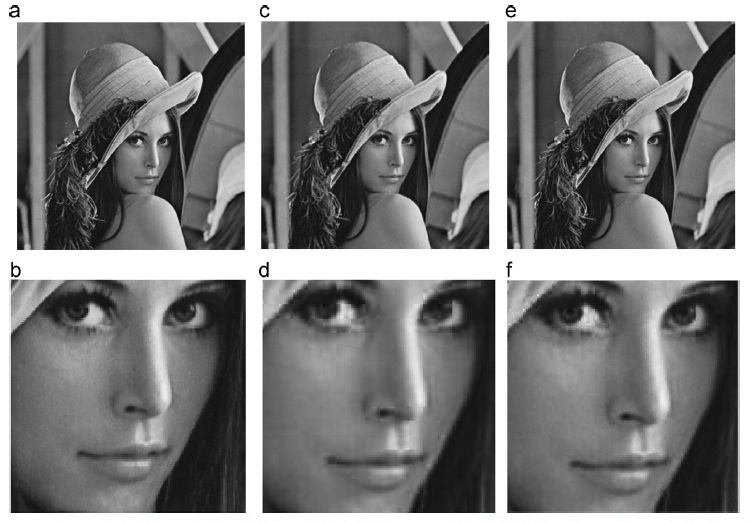# A Fast Hybrid Jacket-Hadamard Matrix Based Diagonal Block-wise Transform

•  Lee, M.H.; Khan, M.H.A.; Kim, K.J.; Park, D., "A Fast Hybrid Jacket-Hadamard Matrix Based Diagonal Block-wise Transform", Image Communication, , January 2014.
• @article{Lee2013dec,
• author = {Lee, M.H. and Khan, M.H.A. and Kim, K.J. and Park, D.},
• title = {A Fast Hybrid Jacket-Hadamard Matrix Based Diagonal Block-wise Transform},
• journal = {Image Communication},
• year = 2014,
• doi = {10.1016/j.image.2013.11.002},
• url = {https://www.merl.com/publications/TR2014-002}
• }
• ##### Research Area:Image coding results showing DCT-II only and jointly optimized DCT/DST-II compression. (a) Original Lean image, (b) zoomed original Lena image, (c) DCT-II compressed Lena image (PSNR=32.38db), (d) zoomed DCT-II compressed Lena image, (e) DCT/DST-II compressed Lena image (PSNR=35.12db) and (f) zoomed DCT/DST-II compressed Lena image.

In this paper, based on the block (element)-wise inverse Jacket matrix, a unified fast hybrid diagonal block-wise transform (FHDBT) algorithm is proposed. A new fast diagonal block matrix decomposition is made by the matrix product of successively lower order diagonal Jacket matrix and Hadamard matrix. Using a common lower order matrix in the form of 1 1, a fast recursive structure can be developed in the FHDBT, which is able to convert a newly developed discrete cosine transform (DCT)-II, discrete sine transform (DST)-II, discrete Fourier transform (DFT), and Haar-based wavelet transform (HWT). Since these DCT-II, DST-II, DFT, and HWT are widely used in different areas of applications, the proposed FHDBT can be applied to the heterogeneous system requiring several transforms simultaneously. Comparing with pre-existing DCT-II, DST-II, DFT, and HWT, it is shown that the proposed FHDBT exhibits less the complexity as its matrix size gets larger. The proposed algorithm is also well matched to circulant channel matrix. From the numerical experiments, it is shown that a better performance can be achieved by the use of DCT/DST-II compression scheme compared with the DCT-II only compression method.